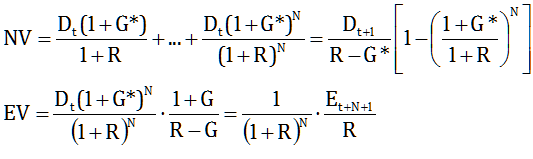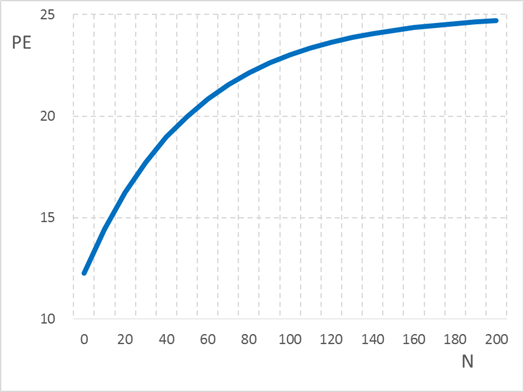In a one-period valuation model the company grows at a constant rate, now and forever, and its Franchise Value – if it has one – is based on a constant and permanent spread between its return on equity and its cost of capital. This is unlikely for most companies: the franchise spread has a finite life and the ROE eventually converges to, and possibly past R.

This is the reason why a more realistic valuation model needs to allow for a period, from 0 to N, in which the company uses its franchise, invests at ROE>R and grows at G*=H*∙ROE, where H* can approximate 1 (hence G*≈ROE) as, in order to maximise growth opportunities, the company reinvests most of its earnings in the business. After that, having exhausted its franchise, the company can continue to grow, but at the lower rate G=H∙R:The company’s valuation can thus be decomposed into an N-period Value NV and a Terminal, or End Value EV:Notice that, unlike the one-period model, where, in order to prevent a negative valuation, R must be larger than G, the two-period model allows for G* to exceed R, as the two factors in NV have the same sign: the first factor is the value of a company that grows at G* forever; the second factor truncates that growth to N periods. After N periods, EV is the present value of a company growing from then onwards at G. Equivalently, it is the present value of the Tangible Value at t+N. Notice that EV also depends on G*, as G* determines the level of earnings from which the company will continue to grow at G after the N-period.

As an example, let’s say ROE=15% and H*=90%, hence G*=13.5%, and N=10. With normalised earnings E=4 and cost of capital R=8% we have NV=23.4. After the N-period, ROE drops to 8% and the franchise spread disappears. Then, assuming that H is reduced to 50%, we have G=4% and therefore EV=75.3, for a total valuation of 98.7 and a PE ratio on normalised earnings of 24.7 (a spreadsheet to play with the numbers is available upon request).

Notice that the N-period value NV is not the same as the Franchise Value FV in the one-period model. If we set ROE=R=8% and H*=H=50%, hence G*=G=4%, the company’s valuation drops to 50, which is equal to the Tangible Value in the one-period model, as FV=0. But in the two-period model NV=15.7 and EV=34.3. NV is simply the portion of the company’s valuation pertaining to the N-period, whether or not G* is greater than G. The Franchise Value in the two-period model is equal to the difference between the company’s valuation and the Tangible Value. In our example, TV=50 and therefore FV=48.7. FV depends on the franchise spread in the N-period and on the length of the period. For example, raising N from 10 to 20 almost triples FV to 135.6, as the company has 10 more years to grow at G*.

Notice that a growth of 13.5% could not even be contemplated in the one-period model, as it would exceed the cost of capital. If we take a different example, with ROE=12% and H*=50%, so that G*=6%<R, as we saw in the previous post we have TV=50 and FV=50, hence a total valuation of 100, with base PE=12.5 and premium PE=12.5, hence a normalised PE ratio of 25. But that is because in the one-period model the franchise spread is assumed to last forever. In the two-period model, where the spread lasts for N years, the Franchise Value for N=10 is only 7.7, for a total valuation of 57.7 and a PE ratio of 14.4. For N=20 the valuation is 64.9 and the PE is 16.2. As shown in the following graph, the PE ratio starts from the 12.5 base and tends to 25 as N grows, but it is a lot lower for realistic values of N.So, while the Tangible Value and the base PE can emerge even from a simple one-period model, in order to obtain a sensible Franchise Value we need a two-period model. If we want to be over meticulous, we could even have a three-period model, where in the middle period ROE declines gradually towards R. But we already have so many uncertain parameters to play with that the additional complication is most probably not worth the candle.

The DDR model is also a two-period model. In our DDR example, we had a 10-year stream of forecasted dividends (2, 4, 4, 4, 5, 5, 5, 6, 6, 7), R=5% and G=3%. At a market price of 100, the DDR was 16.1%, and we saw that it was much more sensitive to the terminal assumptions than to the dividend dynamics within the forecast period. In fact, what matters in the N-period is not the set of point estimates, but the annual growth rate between the first and the last dividend, which in our example is 14.9%. Assuming that dividends grow at that rate over the N-period, we get a DDR of 15.6%. This is a small difference in the context of the overall uncertainty surrounding the model’s parameters: increasing the final dividend to 7.3 would be enough to get back to 16.1%.

If we use these parameters in our two-period model, we have G*=14.9% (it doesn’t matter with which combination of ROE and H*), N=10, R=5% and G=3%, which require H=60% and therefore E=5. Hence NV=29.6 and EV=221.3, for a total valuation of 250.9 and a PE ratio of 50.2. The high valuation is due to the small gap between R and G. To work with more realistic numbers, let’s go back to our previous example, where the gap is twice as large and the total valuation is 98.7, with a PE ratio of 24.7. Using the same inputs in the DDR model and a market price of 100 – close to the company’s valuation – we get DDR=7.5%. With the price at exactly 98.7 we get DDR=7.6%, which is the same DDR that we get with G*=G=4% and a price of TV=50. At that price, however, G*=13.5% implies DDR=16.3%

So our two-period model and the DDR model are basically the same thing: there is a one-to-one correspondence between them. But a valuation gap is a more informative output: the company is nearly fairly valued at 100 and 49% undervalued at 50 – this is a clearer message than a DDR level of 7.5% and 16.3%, both in absolute and relative terms. Also, rather than dallying with point estimates, our model focuses on the two key inputs to the Franchise Value: G* and N. And while G* is implicit in the first and last point estimate in the DDR model, when the model is used to compare valuations across companies N is typically fixed, usually at 5 or 10 years. This is an undesirable rigidity, as companies can have significantly different franchise periods. As we have seen in our example, increasing N from 10 to 20 raises the valuation to 185.6 and the normalised PE to 46.4: a company is much more valuable if it can invest at a 15% ROE for 10 more years.

Again, by changing the model’s parameters we can get any valuation we want. But relating the valuation to the parameters helps us explicate our assumptions. A company selling at a normalised PE of 25 is very cheap if it can invest at 15% for 20 years, but fairly priced if it can do so for 10 years. On the other hand, if the ROE is 12%, the fair PE is 20.4 at 10 years and 31.5 at 20 years.

Which is which? Who knows – I guess it’s a moat question. But one thing is for sure: if the market is pricing a good company at close to its base PE, it is not giving any value to the franchise. Now that’s a pearl.

This site uses Akismet to reduce spam. Learn how your comment data is processed.